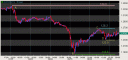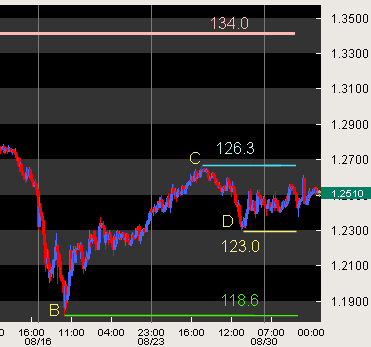Search IntMath
Close

450+ Math Lessons written by Math Professors and Teachers

5 Million+ Students Helped Each Year

1200+ Articles Written by Math Educators and Enthusiasts

Simplifying and Teaching Math for Over 23 Years

# Fibonacci is alive and well - in AUD:SGD

By Murray Bourne, 03 Sep 2007

Observations show that the amount of a market move tends to follow a pattern of:

• Move a lot
• Retrace a bit
• Move another lot - or retrace again

The amount of each move tends to follow Fibonacci retracement levels:

• 0.382
• 0.500
• 0.618

The market volatility over the last few weeks (due to the sub-prime mortgage debacle in the US) has surprised a lot of market watchers. The volatility has been felt by all types of markets, including forex (foreign exchange).

Let's look at an example which how Fibonacci retracement levels can be observed in the recent turmoil.

## SGD-AUD and Fibonacci

Here is a chart of the Australian dollar against the Singapore dollar for the last 30 days (end July to early September 2007. Click to enlarge.)Let's zoom in on the latter part of the chart to see what is happening.The peak of 134.0 (point A - the pink line) was reached on 26th July and the trough (point B) was around 118.6 on 17th August. That's a huge 15.4c drop.

Now, 0.5 × 15.4 = 7.7c

Observe that the AUD:SGD rate retraced to 126.3 (point C) on the 27th August was right on the 0.500 Fibonacci retracement level

118.6 + 0.5 × 15.4 = 118.6 + 7.7 = 126.3

Finally, Point D (29th August) is at 123.0.

Half of the previous rise is 0.5 × 7.7 = 3.85.

126.3 − 3.85 = 122.45. Pretty close to the actual figure of 123.0.

As mentioned above, other possible multiples are 0.382 and 0.618 times the previous move. Traders use these figures to make buy and sell orders in the hope of making money (see Fibonacci method in Forex charts and Money Charts and Fibonacci.).

The good traders would have done very well over the last month. They love extreme volatility.

Be the first to comment below.

### Comment Preview

HTML: You can use simple tags like <b>, <a href="...">, etc.

To enter math, you can can either:

1. Use simple calculator-like input in the following format (surround your math in backticks, or qq on tablet or phone):
a^2 = sqrt(b^2 + c^2)
(See more on ASCIIMath syntax); or
2. Use simple LaTeX in the following format. Surround your math with $$ and $$.
$$\int g dx = \sqrt{\frac{a}{b}}$$
(This is standard simple LaTeX.)

NOTE: You can mix both types of math entry in your comment.